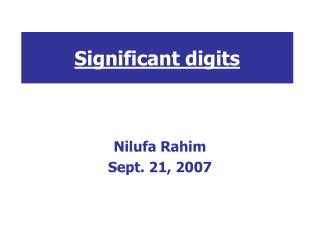DownloadDownload PresentationSignificant digits

# Significant digits

Télécharger la présentation## Significant digits

- - - - - - - - - - - - - - - - - - - - - - - - - - - E N D - - - - - - - - - - - - - - - - - - - - - - - - - - -
##### Presentation Transcript

1. Significant digits Nilufa Rahim Sept. 21, 2007

2. Identifying significant digits • 1. All non-zero digits are significant. Example:'123.45'has five significant figures: 1,2,3,4 and 5. • 2. Zeros appearing in between two other significant digits are significant. Example:'101.12' has five significant figures: 1,0,1,1,2.

3. Identifying significant digits • 3. All final zeros after the decimal point are significant. Example:'12.2300' has six significant figures: 1,2,2,3,0 and 0. The number '0.00122300' still only has six significant digits (the zeros before the '1' are not significant, they are place holders). 8,000.000000 has ten significant digits.

4. Identifying significant digits Identifying significant digits • 4. All zeros appearing in a number without a decimal point and to the right of the last non-zero digit are not significant unless indicated by a bar. Example:'1300' has two significant figures: 1 and 3. The zeros are not considered significant because they don't have a bar. However, 1300.0 has five significant figures.

5. Adding and Subtracting • RULE:When adding or subtracting your answer can only show as many decimal places as the measurement having the fewest number of decimal places. • Example: 3.76 g + 14.83 g + 2.1 g = 20.69 g • Note that 2.1 shows the least number of decimal places. We must round off our answer, 20.69, to one decimal place (the tenth place). Our final answer is 20.7 g

6. Multiplying and Diving • RULE:When multiplying or dividing, the product or quotient should be rounded to the least number of significant digits in the measurement. • Example: 3.22 cm x 2.1 cm = 6.762 cm2 • 3.22 shows 3 significant digits. • 2.1 shows 2 significant digits.

7. Multiplying and Dividing • Our answer can only show 2 significant digits because that is the least number of significant digits in the original problem. • 6.762 shows 4 significant digits, we must round to the tenth place in order to show only 2 significant digits. Our final answer becomes 6.8 cm2.

8. PRACTICE • http://www.lon-capa.org/~mmp/applist/sigfig/sig.htm • http://www.fordhamprep.org/gcurran/sho/sho/lessons/lesson23.htm

9. Thank you